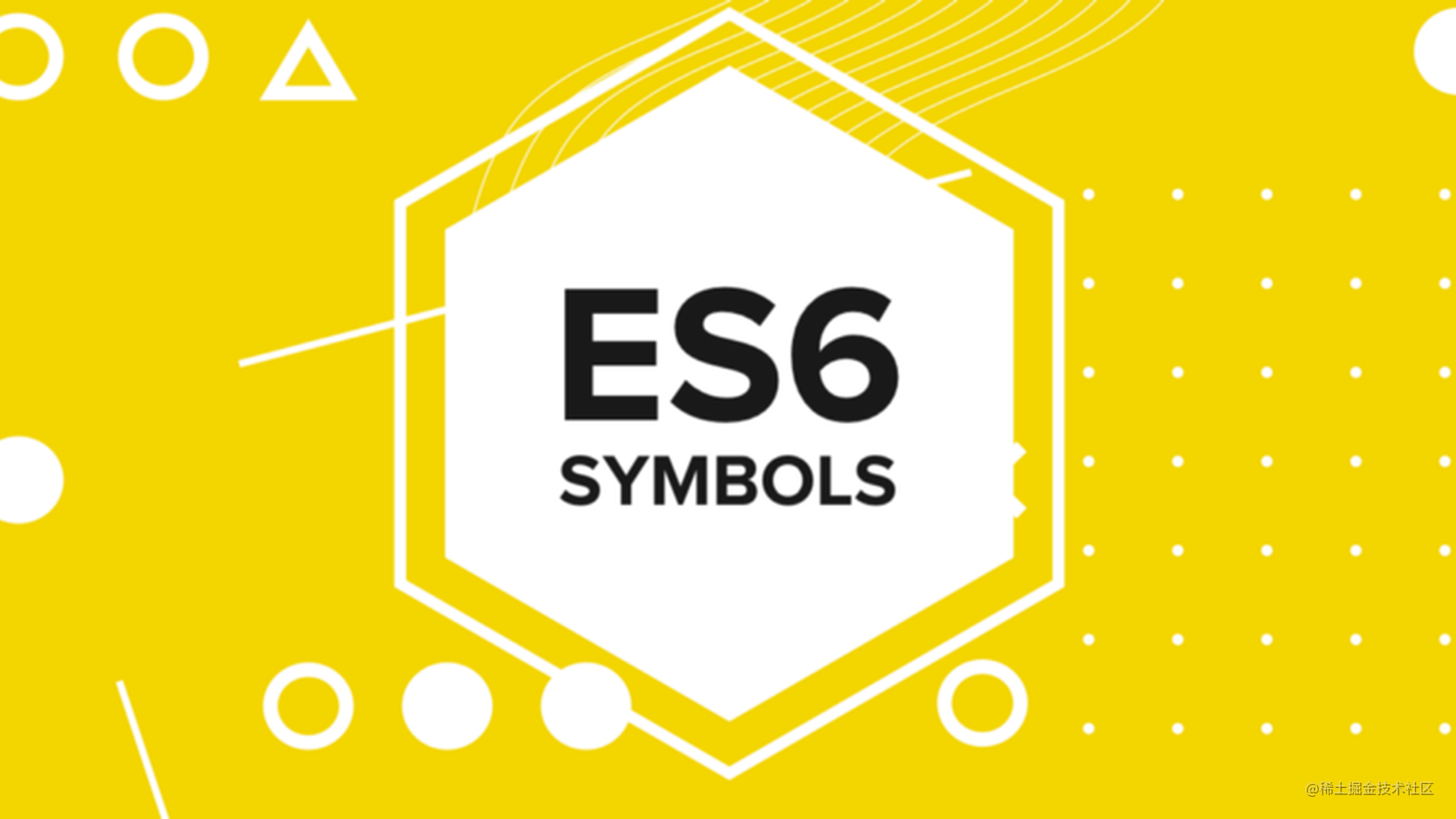# JavaScript ES6 Symbol 终极教程

## 创建Symbol

ES6新增了`Symbol`作为原始数据类型，和其他原始数据类型，像number、boolean、null、undefined、string，symbol类型没有文字形式。

``````let s = Symbol('foo');

`Symbol()` 函数每次调用会创建一个新的唯一值

``````console.log(Symbol() === Symbol()); // false

`Symbol()` 函数接受一个可选参数作为描述，这样使Symbol更具有语义性。

``````let firstName = Symbol('first name'),
lastName = Symbol('last name');

``````console.log(firstName); // Symbol(first name)
console.log(lastName); // Symbol(last name)

``````console.log(typeof firstName);
// symbol

``````let s = new Symbol(); // error

## 共享 symbol

`Symbol.for()` 也接受一个可选参数作为描述

``````let ssn = Symbol.for('ssn');

`Symbol.for()` 会首先在全局中查找是否有已经创建的`ssn`的symbol，如果有就会返回已经创建的symbol，如果没有就会创建一个新的symbol。

``````let nz = Symbol.for('ssn');
console.log(ssn === nz); // true

``````console.log(Symbol.keyFor(nz)); // 'ssn'

``````let systemID = Symbol('sys');
console.log(Symbol.keyFor(systemID)); // undefined

## Symbol 有啥用

### 一) 使用Symbol作唯一值

``````let statuses = {
OPEN: Symbol('已下单'),
IN_PROGRESS: Symbol('配送中'),
COMPLETED: Symbol('订单完成'),
CANCELED: Symbol('订单取消')
};

// 完成订单

### 二) 使用 symbol 作为对象属性

``````let status = Symbol('status');

[status]: statuses.OPEN,
description: '学习 ES6 Symbol'
};

``````console.log(Object.keys(task));
// ["description"]

``````console.log(Object.getOwnPropertyNames(task));
// ["description"]

``````console.log(Object.getOwnPropertySymbols(task));
//[Symbol(status)]

## Well-known symbol

ES6在原型链上定义了与 `Symbol` 相关的属性来暴露更多的语言内部逻辑。`well-known Symbol` 为标准对象定义了一些以前只在语言内部可见的功能。

### Symbol.hasInstance

`Symbol.hasInstance` 是一个改变`instanceof`操作符默认行为的symbol，通常我们会这样使用`instanceof`

``````obj instanceof type;

``````type[Symbol.hasInstance](obj);

``````class Stack {
}
console.log([] instanceof Stack);
// false

`[]` 数组不是Stack类所创建的实例，所以返回false。

``````class Stack {
static [Symbol.hasInstance](obj) {
return Array.isArray(obj);
}
}
console.log([] instanceof Stack);
// true

### Symbol.iterator

`Symbol.iterator` 指定函数是否会返回对象的迭代器。

ES6提供了for...of循环，它可以用在可迭代对象上。

``````var numbers = [1, 2, 3];
for (let num of numbers) {
console.log(num);
}

// 1
// 2
// 3

``````var iterator = numbers[Symbol.iterator]();

console.log(iterator.next()); // Object {value: 1, done: false}
console.log(iterator.next()); // Object {value: 2, done: false}
console.log(iterator.next()); // Object {value: 3, done: false}
console.log(iterator.next()); // Object {value: undefined, done: true}

``````class List {
constructor() {
this.elements = [];
}

this.elements.push(element);
return this;
}

*[Symbol.iterator]() {
for (let element of this.elements) {
yield element;
}
}
}

let chars = new List();

// 使用Symbol.iterator实现了迭代
for (let c of chars) {
console.log(c);
}

// A
// B
// C

``````let odd  = [1, 3],
even = [2, 4];
let all = odd.concat(even);
console.log(all); // [1, 3, 2, 4]

``````let extras = all.concat(5);
console.log(extras); // [1, 3, 2, 4, 5]

``````let list = {
0: 'JavaScript',
1: 'Symbol',
length: 2
};
let message = ['Learning'].concat(list);
console.log(message); // ["Learning", Object]

``````let list = {
0: 'JavaScript',
1: 'Symbol',
length: 2,
};
let message = ['Learning'].concat(list);
console.log(message); // ["Learning", "JavaScript", "Symbol"]

### Symbol.toPrimitive

`Symbol.toPrimitive` 方法决定了当一个对象被转换成原始值时的行为。

JavaScript引擎在每个类型值的原型上定义了Symbol.toPrimitive方法。

`Symbol.toPrimitive`方法接受一个hint参数，该参数会是下面三个值，string、number、default，hint参数用来指定返回值的类型。hint参数由JavaScript引擎根据使用对象的上下文进行填充。

``````function Money(amount, currency) {
this.amount = amount;
this.currency = currency;
}

Money.prototype[Symbol.toPrimitive] = function(hint) {
var result;
switch (hint) {
case 'string':
result = this.amount + this.currency;
break;
case 'number':
result = this.amount;
break;
case 'default':
result = this.amount + this.currency;
break;
}
return result;
}

var price = new Money(10000, '人民币');

console.log('我有 ' + price); // Price is 799USD
console.log(+price + 1); // 800
console.log(String(price)); // 799USD

### 其他

• `Symbol.match(regex)`：一个在调用 `String.prototype.match()` 方法时调用的方法，用于比较字符串。

• `Symbol.replace(regex, replacement)`：一个在调用 `String.prototype.replace()` 方法时调用的方法，用于替换字符串的子串。

• `Symbol.search(regex)`：一个在调用 `String.prototype.search()` 方法时调用的方法，用于在字符串中定位子串。

• `Symbol.species(regex)`：用于创建派生对象的构造函数。

• `Symbol.split`：一个在调用 `String.prototype.split()` 方法时调用的方法，用于分割字符串。

• `Symbol.toStringTag`：一个在调用 `String.prototype.toString()` 方法时使用的字符串，用于创建对象描述。

• `Symbol.unscopables`：一个定义了一些不可被 with 语句引用的对象属性名称的对象集合。